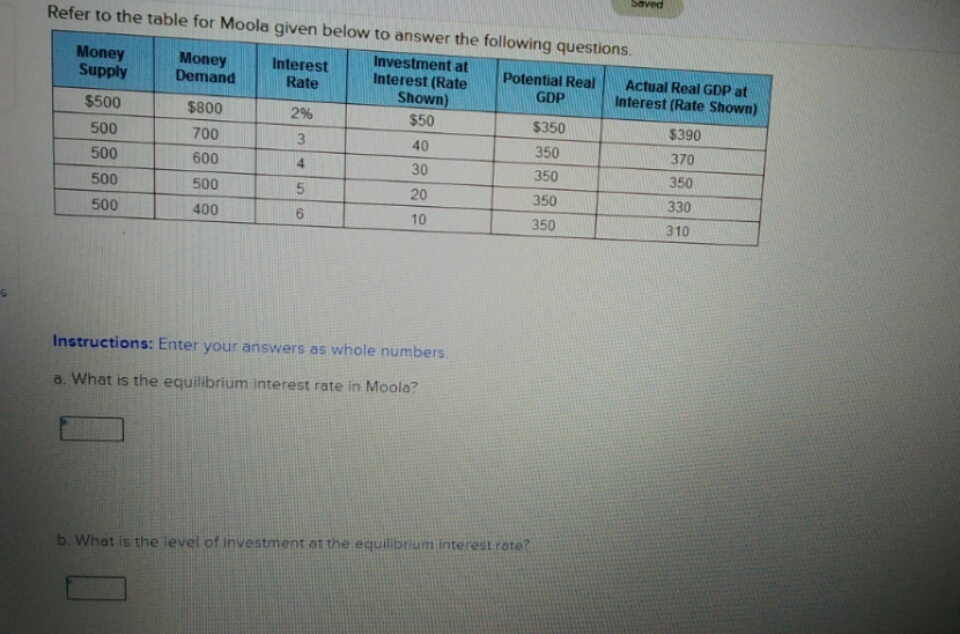# Refer to the table for Moola given below to answer the following questionsInvestment atInterest (RateShown)MoneySupplyMoneyDemandInterestRatePotential RealActual Real GDP atInterest (Rate Shown)GDP\$500\$8002%\$50\$350\$390500700403503705006003035035050050052035033040050010350310Instructions: Enter your answers as whole numbersa. What is the equilibrium interest rate in Moola?b.What is the level of investment at the equilibrium interest rate?

Question
874 views

c) Is there either a recessionary output gap (negative GDP gap) or an inflationary output gap (positive GDP gap) at the equilibrium interest rate, and, if either, what is the amount?

Given money demand, by how much would the Moola central bank need to change the money supply to close the output gap?

What is the expenditure multiplier in Moola?help_outlineImage TranscriptioncloseRefer to the table for Moola given below to answer the following questions Investment at Interest (Rate Shown) Money Supply Money Demand Interest Rate Potential Real Actual Real GDP at Interest (Rate Shown) GDP \$500 \$800 2% \$50 \$350 \$390 500 700 40 350 370 500 600 30 350 350 500 500 5 20 350 330 400 500 10 350 310 Instructions: Enter your answers as whole numbers a. What is the equilibrium interest rate in Moola? b.What is the level of investment at the equilibrium interest rate? fullscreen
check_circle

star
star
star
star
star
1 Rating
Step 1

a. The equilibrium interest rate occurs at the point where the demand for money is equal to the supply of money. The demand for money and supply of money is equal (\$500) at interest rate 5%. Thus, the equilibrium interest rate is 5%.

Step 2

b. Table reveals that the level of investment at 5% interest rate is \$20.

Step 3

c. Output gap can be calc...

### Want to see the full answer?

See Solution

#### Want to see this answer and more?

Solutions are written by subject experts who are available 24/7. Questions are typically answered within 1 hour.*

See Solution
*Response times may vary by subject and question.
Tagged in